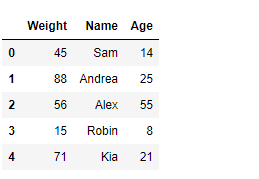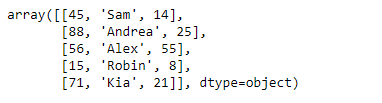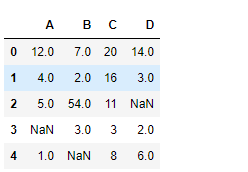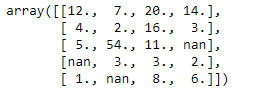# Python | Pandas DataFrame.values

• Last Updated : 20 Feb, 2019

Pandas DataFrame is a two-dimensional size-mutable, potentially heterogeneous tabular data structure with labeled axes (rows and columns). Arithmetic operations align on both row and column labels. It can be thought of as a dict-like container for Series objects. This is the primary data structure of the Pandas.

Pandas` DataFrame.values` attribute return a Numpy representation of the given DataFrame.

Syntax: DataFrame.values

Parameter : None

Returns : array

Example #1: Use `DataFrame.values` attribute to return the numpy representation of the given DataFrame.

 `# importing pandas as pd``import` `pandas as pd`` ` `# Creating the DataFrame``df ``=` `pd.DataFrame({``'Weight'``:[``45``, ``88``, ``56``, ``15``, ``71``],``                   ``'Name'``:[``'Sam'``, ``'Andrea'``, ``'Alex'``, ``'Robin'``, ``'Kia'``],``                   ``'Age'``:[``14``, ``25``, ``55``, ``8``, ``21``]})`` ` `# Print the DataFrame``print``(df)`

Output :Now we will use `DataFrame.values` attribute to return the numpy representation of the given DataFrame.

 `# return the numpy representation of ``# this dataframe``result ``=` `df.values`` ` `# Print the result``print``(result)`

Output :As we can see in the output, the `DataFrame.values` attribute has successfully returned the numpy representation of the given DataFrame.

Example #2: Use `DataFrame.values` attribute to return the numpy representation of the given DataFrame.

 `# importing pandas as pd``import` `pandas as pd`` ` `# Creating the DataFrame``df ``=` `pd.DataFrame({``"A"``:[``12``, ``4``, ``5``, ``None``, ``1``], ``                   ``"B"``:[``7``, ``2``, ``54``, ``3``, ``None``], ``                   ``"C"``:[``20``, ``16``, ``11``, ``3``, ``8``], ``                   ``"D"``:[``14``, ``3``, ``None``, ``2``, ``6``]}) `` ` `# Print the DataFrame``print``(df)`

Output :Now we will use `DataFrame.values` attribute to return the numpy representation of the given DataFrame.

 `# return the numpy representation of ``# this dataframe``result ``=` `df.values`` ` `# Print the result``print``(result)`

Output :As we can see in the output, the `DataFrame.values` attribute has successfully returned the numpy representation of the given DataFrame.

My Personal Notes arrow_drop_up